﻿ 20-sim webhelp > Library > Iconic Diagrams > Mechanical > Translation > Components > Friction-Relative

# Friction-Relative

Navigation:  Library > Iconic Diagrams > Mechanical > Translation > Components >

# Friction-Relative## Library

Iconic Diagrams\Mechanical\Translation\Components

C

V

CV

SCVS

LuGre

## Use

Domains: Continuous. Size: 1-D. Kind: Iconic Diagrams (Translation).

## Introduction

These models represents friction relative to other objects. The amount of friction depends on the normal force that is applied and the friction function that is used. The normal force is given by the input signal Fn.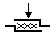The port p of the friction models have separate high and low terminals. The equations are:

p.F = p_high.F = p_low.F

p.v = p_high.v - p_low.v

Due to the use of normal force, the friction models all have a fixed torque out causality. The constitutive equations are therefore described as:

p.F = Fn * f(p.v);

with f the friction function.

## Description  -C

This model represents friction between two terminals described as coulomb friction:

p.F = Fn*mu_c*tanh(slope*p.v);

Fn: normal force (given by the input signal Fn)

mu_c: the coulomb friction coefficient

slope: the steepness of the coulomb friction curve.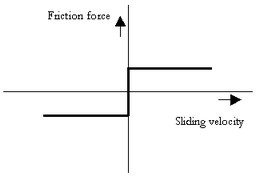## Interface - C

 Ports Description p_high, p_low Both terminals of port p (Translation). Causality Fixed force out Input Fn Normal force [N] Parameters mu_c slope Coulomb friction coefficient [] Steepness of Coulomb friction curve [s/m]

## Description - V

This model represents friction between two terminals described as viscous friction:

p.F = Fn*mu_v*p.v;

Fn: normal force (given by the input signal Fn)

mu_v: the viscous friction coefficient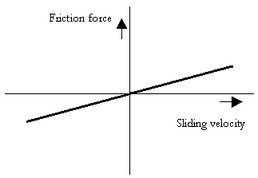## Interface -V

 Ports Description p_high, p_low Both terminals of port p (Translation). Causality Fixed force out Input Fn Normal force [N] Parameters mu_v Viscous friction coefficient [s/m]

## Description - CV

This model represents friction between two terminals described as coulomb plus viscous friction:

p.F = Fn*(mu_c*tanh(slope*p.v) + mu_v*p.v);

Fn: normal force (given by the input signal Fn)

mu_v: the viscous friction coefficient

mu_c: the coulomb friction coefficient

slope: the steepness of the coulomb friction curve.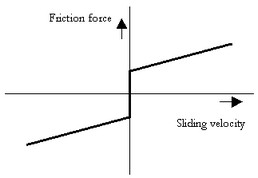## Interface - CV

 Ports Description p_high, p_low Both terminals of port p (Translation). Causality Fixed force out Input Fn Normal force [N] Parameters mu_v mu_c slope Viscous friction coefficient [s/m] Coulomb friction coefficient [] Steepness of Coulomb friction curve [s/m]

## Description - SCVS

This model represents friction between two terminals described as static plus coulomb plus viscous plus Stribeck friction:

p.F = Fn *

(( mu_c + (mu_st*abs(tanh( slope*p.v )) - mu_c)

* exp( -((p.v / v_st)^2 )) ) * sign(p.v)

+ mu_v * p.v);

Fn: normal force (given by the input signal Fn)

mu_s: the static friction coefficient

mu_v: the viscous friction coefficient

mu_c: the coulomb friction coefficient

slope: the steepness of the coulomb and static friction curve.

v_st: the characteristic Stribeck velocity.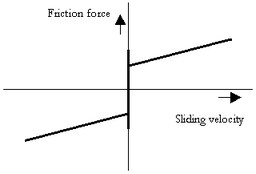## Interface - SCVS

 Ports Description p_high, p_low Both terminals of port p (Translation). Causality Fixed force out Input Fn Normal force [N] Parameters mu_s mu_v mu_c slope v_st Static friction coefficient [] Viscous friction coefficient [s/m] Coulomb friction coefficient [] Steepness of Coulomb friction curve [s/m] Characteristic Stribeck velocity [m/s]

## Description - LuGre

This model represents friction between two terminals described by the LuGre friction model:

p.F = FN*f_lg(p.v);

Fn: normal force (given by the input signal Fn)

f_lg: the LuGre friction model

## Interface - LuGre

Ports

Description

p_high, p_low

Both terminals of port p (Translation).

Causality

Fixed force out

Input

Fn

Normal force [N]

## Parameters

mu_c

mu_s

mu_v

v_st

mu_k

Coulomb friction coefficient []

Static friction coefficient []

Viscous friction coefficient [s/m]

Characteristic Stribeck velocity [m/s]

Stiffness coefficient at zero speed []